# NCERT class 4 maths chapter 6 The Junk Seller

NCERT book Page No: 60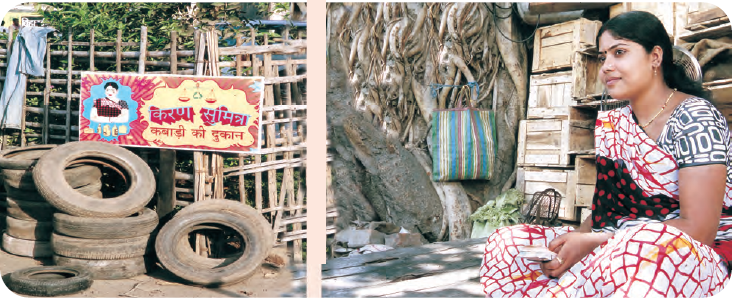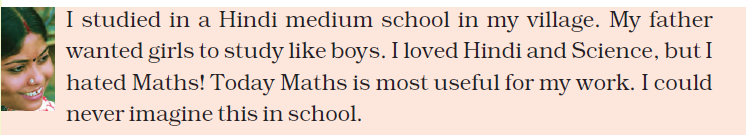Question: 1

(a) What about you? Do you also find Maths difficult?

(b) What is the most difficult thing in your Maths book?

(c) What do you think is the easiest lesson?

(a) No, Maths is not difficult if it is practiced well.

(b) All the topics are easy to me

(c) It depends on the practice. The more you practice, the more it will be easy.

NCERT book Page No: 61

Find out: how much for a cup of tea?

Question: 2

Ask people and find out the cost of a cup of tea

(a) At a tea stall

(b) At a hotel

(a) The cost of tea at a tea stall is Rs 5

(b) The cost of tea at a hotel is Rs 10

Question: 3

(a) If a person who runs a tea stall earns Rs 30 in a day, how much will he earn in 10 days?

(b) And in a month?

(a) Earning in a day = Rs 30 × 1

Hence, earning in two days will be = Rs 30 × 2

Earning in 3 days will be = Rs 30 × 3

Earning in 10 days will be = Rs 30 × 10

= Rs 300

(b) In a month there are 30 days

If earning in a day cost Rs 30

Then, earning in 30 days will cost = Rs 30 × 30

= Rs 900

Question: 4

Find out: what is a loan?

(a) Have you ever heard of someone taking a loan? For what?

(b) How much loan was taken?

(c) How much money was paid back?

(a) Yes, I heard a person who took a loan for his son’s education.

(b) He took Rs 100000

(c) The money paid back by him after one year is Rs 150000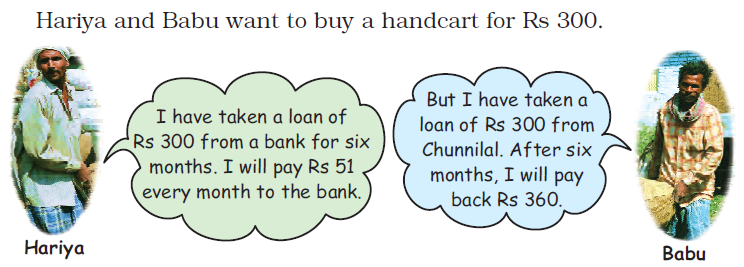Question: 5

Who has to pay back more – Hariya or Babu?

Answer: For six months, Hariya should pay Rs 51

Total money paid by Hariya = Rs 51 × 6

= Rs 306

But Babu pays Rs 360 after six months. Hence, Babu pays more money than Hariya.

NCERT book Page No: 62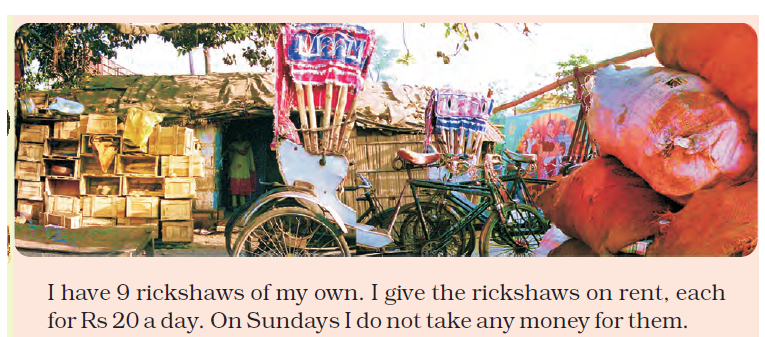Question: 6

How much does Kiran earn from 9 rickshaws in a day?

Answer: From 1 rickshaw she earns = Rs 20

So, from 2 rickshaws she earns = Rs 20 × 2 = Rs 40

Similarly from 9 rickshaws she earns = Rs 20 × 9

= Rs 180

Question: 7

For 10 rickshaws she will get Rs 20 × 10 = Rs 200

So, for 9 rickshaws, she will get Rs 200 – _______ = _______

Answer: For 9 rickshaws she will get Rs 200 – 20

= Rs 180

NCERT book Page No: 63

Question: 8

In a week how much does Kiran earn from one rickshaw?

Answer: We know in a week, there are 7 days

Money earned by Kiran in a day = Rs 20

Hence, money earned by Kiran in a week from one rickshaw = Rs 20 × 7

= Rs 140

Question: 9

Do it mentally and write the answers.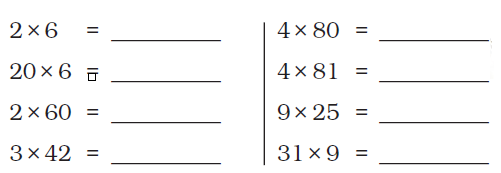2 × 6 = 12

20 × 6 = 120

2 × 60 = 120

3 × 42 = 126

4 × 80 = 320

4 × 81 = 324

9 × 25 = 225

31 × 9 = 279

How Much to Pay for this Junk?

Kiran has bought some junk from junk collectors

Question: 10

How much will Kiran pay for 31 kg newspaper?

Answer: 1 kg newspaper cost = Rs 5

31 kg newspaper cost = Rs 5 × 31 = Rs 155

Hence, the cost of 31 kg newspaper is Rs 155

NCERT book Page No: 64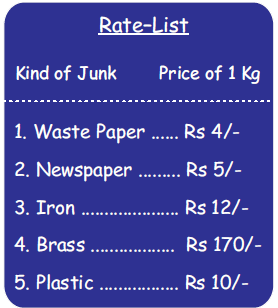Question: 11

How much will Kiran pay for 42 kg newspaper?

Answer: For 1 kg newspaper Kiran pays = Rs 5

For 42 kg of newspaper she will pay = Rs 5 × 42

= Rs 210

Hence, Kiran pays Rs 210 for 42 kg of newspaper.

Question: 12

Also find the cost of:

(a) 22 kg of plastic

(b) 23 kg of waste paper

(c) 12 kg of iron

(a) The cost of 1 kg of plastic = Rs 10

So, the cost of 22 kg of plastic will be = Rs 10 × 22

= Rs 220

(b) Rate of 1 kg of waste paper = Rs 4

Hence, the cost of 23 kg of waste paper = Rs 4 × 23

= Rs 92

(c) The cost of 1 kg of iron = Rs 12

Hence, the cost of 12 kg of iron will be = Rs 12 × 12

= Rs 144

Question: 13

Guess the total money Kiran will pay to the junk collectors. Will it be

– More than 600

– Less than 600?

Answer: Total money Kiran pays to the junk collectors are

Cost of 42 kg of newspaper = Rs 210

Cost of 22 kg of plastic = Rs 220

Cost of 23 kg of waste paper = Rs 92

Cost of 12 kg of iron = Rs 144

Therefore, total cost of all the junks = Rs 666

So, Kiran pays more than Rs 600

NCERT book Page No: 64 – 66

Smart Kiran Sells the Junk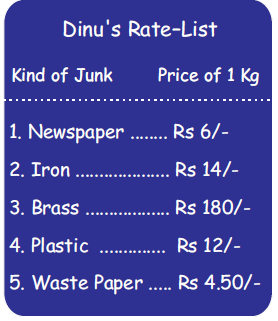Question: 14

Kiran bought 1 kg plastic for Rs 10, but sold 1 kg plastic for Rs 12. How much money does she earn on selling 1 kg plastic?

Answer: The cost of 1 kg of plastic that Kiran bought = Rs 10

She sold 1 kg of plastic for Rs 12

Hence, she earned = Rs 12 – Rs 10

= Rs 2

Question: 15

So, how much money does she earn for 63 kg?

Answer: She earns Rs 2 for 1 kg of plastic

For 63 kg of plastic she earns = Rs 2 × 63

= Rs 126

Therefore, Kiran earns Rs 126 for 63 kg of plastic

This can be calculated as follows

 60 3 2 60 × 2 3 × 2 120 6

By adding the digits in last row we will get 126

Kiran sells 32 kg iron

Question: 16

How much money will Dinu pay for 32 kg iron?

Answer: The amount by which 1 kg of plastic is sold = Rs 14

Hence, the cost of 32 kg of plastic = Rs 14 × 32

= Rs 448

This can be calculated as follows

 30 2 10 30 × 10 2 × 10 300 20 4 30 × 4 2 × 4 120 8

By adding the bold numbers, we get the desired number

300 + 20 + 120 + 8 = 448

Hence, the money paid by Dinu for 32 kg of plastic is Rs 448

Question: 17

Kiran buys 1 kg iron for Rs 12, but sells it for Rs 14. How much does she earn when she sells 32 kg of iron?

Answer: Money earned by Kiran by selling 1 kg of iron = Rs 14 – Rs 12

= Rs 2

Hence, money earned by her by selling 32 kg of iron = Rs 2 × 32

= Rs 64

This can be calculated as follows:

 30 2 2 30 × 2 2 × 2 60 4

By adding the numbers in last row, we can find the desired number

60 + 4 = 64

Therefore, by selling 32 kg of iron, Kiran earn Rs 64

What will Dinu pay for 152 kg newspaper?

Question: 18

I bought 1 kg newspaper for Rs 5, but sold it for Rs 6. How much money did I earn by selling 152 kg of newspaper?

Answer: Money earned by selling 1 kg of newspaper = Selling price of newspaper – Purchasing price of newspaper

= Rs 6 – Rs 5

= Rs 1

Money earned by her by selling 152 kg of newspaper = Rs 1 × 152

= Rs 152

Therefore, she earns Rs 152 by selling 152 kg of newspaper

What does Dinu pay for brass?

Question: 19

How much money will Dinu pay for 4 kg brass?

Answer: As per the Dinu’s price list, the cost of 1 kg of brass = Rs 180

For 4 kg of brass, Dinu will pay = Rs 180 × 4

= Rs 720

This can also be calculated as follows:

 100 80 4 100 × 4 80 × 4 400 320

We get the desired result by adding the digits in last row

400 + 320 = 720

Hence, for 4 kg brass Dinu pays Rs 720

NCERT book Page No: 67

Question: 20

First guess the answer and then calculate:

(a) 37 × 18 =

(b) 45 × 24 =

(c) 69 × 52 =

(d) 77 × 55 =

(e) 142 × 5 =

(f) 382 × 3 =

(g) 2 × 175 =

(h) 4 × 206 =

(a) Here, the number will be near to 40 × 20 i.e., about 800

Calculation:

 30 7 10 30 × 10 7 × 10 300 70 8 30 × 8 7 × 8 240 56

Now add the digits in third and fifth row, we get

300 + 70 + 240 + 56 = 666

Therefore, 37 × 18 = 666

(b) 45 × 24

This number will be near to 40 × 25 = 1000

Calculation:

 40 5 20 40 × 20 5 × 20 800 100 4 40 × 4 5 × 4 160 20

Add the digits in third and fifth row, we get

800 + 100 + 160 + 20 = 1080

Hence, 45 × 24 = 1080

(c) 69 × 52

Here the number will be near to 70 × 50 = 3500

Calculation:

 60 9 50 60 × 50 9 × 50 3000 450 2 60 × 2 9 × 2 120 18

On adding the digits in third and fifth row, we get

3000 + 450 + 120 + 18 = 3588

Hence, 69 × 52 = 3588

(d) By guessing 77 × 55

The number will be near to 80 × 50 = 4000

Calculation:

 70 7 50 70 × 50 7 × 50 3500 350 5 70 × 5 7 × 5 350 35

On adding the numbers in third and fifth row, we get

3500 + 350 + 350 + 35 = 4235

Therefore, 77 × 35 = 4235

(e) On guessing 142 × 5

The number will be near to 140 × 5 = 700

Calculation:

 100 40 2 5 100 × 5 40 × 5 2 × 5 500 200 10

By adding the numbers in last row, we get

500 + 200 + 10 = 710

Therefore, 142 × 5 = 710

(f) 382 × 3

The number will be near to 380 × 3 = 1140 on guessing

Calculation:

 300 80 2 3 300 × 3 80 × 3 2 × 3 900 240 6

On adding the digits in last row, we will get

900 + 240 + 6 = 1146

So, 382 × 3 = 1146

(g) 2 × 175

On guessing this number will be near to 2 × 175 = 350

Calculation:

 100 70 5 2 100 × 2 70 × 2 5 × 2 200 140 10

On adding the digits in last row, we get

200 + 140 + 10 = 350

(h) 4 × 206

This number will be near to 5 × 200 = 1000 on guessing

Calculation:

 200 6 4 200 × 4 6 × 4 800 24

On adding the digits in last row, we get

800 + 24 = 824

Therefore, 4 × 206 = 824

Fill My Diary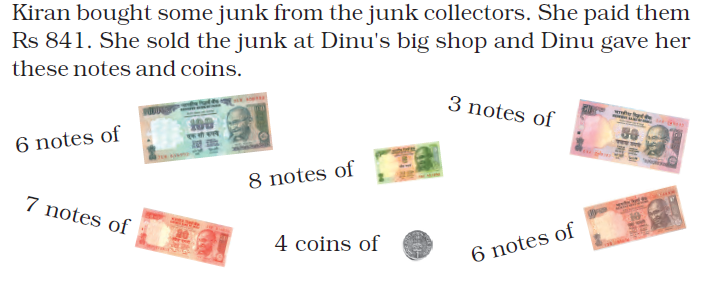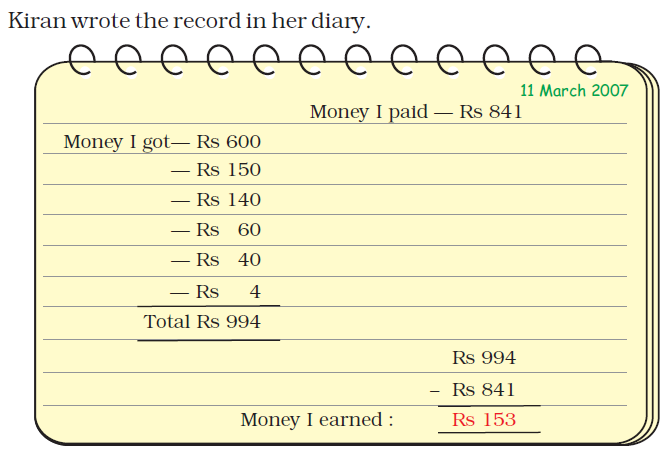Later she paid Rs 919 to the junk collectors. When she sold the junk she got these notes and coins from Dinu.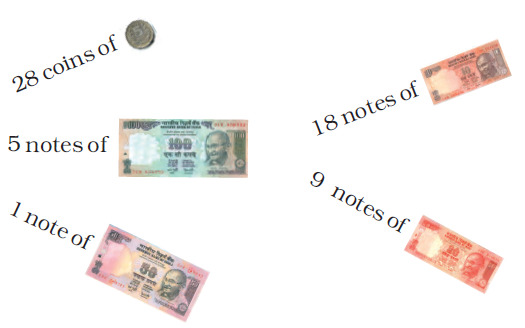Question: 21

Find out how much she earned this time.

 18 March 2007 Money paid by Kiran = Rs 919 Money she got from Dinu Rs 100 × 5 = Rs 500 Rs 50 × 1 = Rs 50 Rs 20 × 9 = Rs 180 Rs 10 × 18 = Rs 180 Rs 5 × 28 = Rs 140 Total money she got from Dinu = Rs 1050 Rs 1050 – Rs 919 ___________ Total money she earned = Rs 131 = Rs 131 ___________

NCERT Class 4 maths chapter 6 covers the topic – The junk seller. Chapter-wise syllabus helps to revise the concepts at your speed and practice to perfection. NCERT publishes textbooks prescribed by the Central Board Of Secondary Education (CBSE). BYJU’S offers a platform for students with all the study materials such as textbooks, notes, question papers, etc.

To provide good practice and detailed understanding of each concept, CBSE class 4 maths worksheets are also provided here, which gives a thorough insight of thoughts explained in the chapter, such as The Junk Seller. Below are some of the example questions;

Q1. Do it mentally:

1. 3 x 81 = ____
2. 10 x 6 = ____

1. 4 x 81 is 4 more than 4 x 80?

Also, solve some more maths questions related to this chapter, The junk seller and get your doubts clear with the help of solved examples.

Download BYJU’S-The Learning App and get interactive and interesting videos explaining the concepts and fundamental present in Maths and Science subject.

## Frequently Asked Questions on NCERT Solutions for Class 4 Maths Chapter 6

### In Chapter 6 of NCERT Solutions for Class 4 Maths, out of Hariya and Babu who had to pay back more for the loan they took individually?

Both Hariya and Babu took a loan individually for Rs 300. Hariya had to pay Rs 51 every month back to the bank for six months. So, Hariya will pay back Rs 306 for the loan he took. Now, in the case of Babu, he had to pay back Rs 360 to Chunnilal after six months. Clearly, Babu had to pay more as compared to Hariya.

### How much does Kiran earn from her rickshaws in a day according to chapter 6 of NCERT Solutions for Class 4 Maths?

Kiran has 9 rickshaws of her own and gives them on rent, each for Rs 20 a day. Hence, for 9 rickshaws Kiran earns (Rs 9 x 20) i.e. Rs 180.

### How does Kiran make money by selling junks as discussed in Chapter 6 of NCERT Solutions for Class 4 Maths?

Kiran first buys some junk from junk collectors. She has her own rate-list for which she buys those junk. Later, Kiran sells her junk to a big shop. She checks the prices on her mobile phone and sells only when she gets a good price.